# Sample Data: Leukaemia NW England

Locations of leukaemia in N.W. England from 1982 to 1998 (inclusive), annotated with age, gender, deprivation index (higher values indicating less affluence), and subject type (case or control) marks

## Details

Locations of leukaemia in N.W. England from 1982 to 1998 (inclusive) in the observation region that is the polygon of N.W. England, annotated with age, gender, deprivation index (higher values indicating less affluence), and subject type (case or control) marks.

## Examples

### Basic Examples (2)

Retrieve the data:

 In:=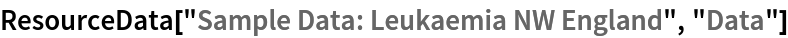Out=Summary of the spatial point data:

 In:=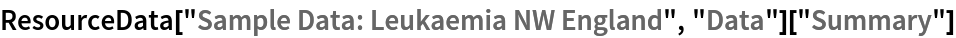Out=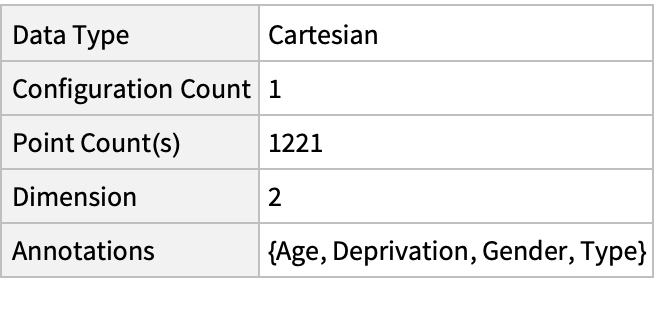### Visualizations (3)

Plot the spatial point data:

 In:=Out=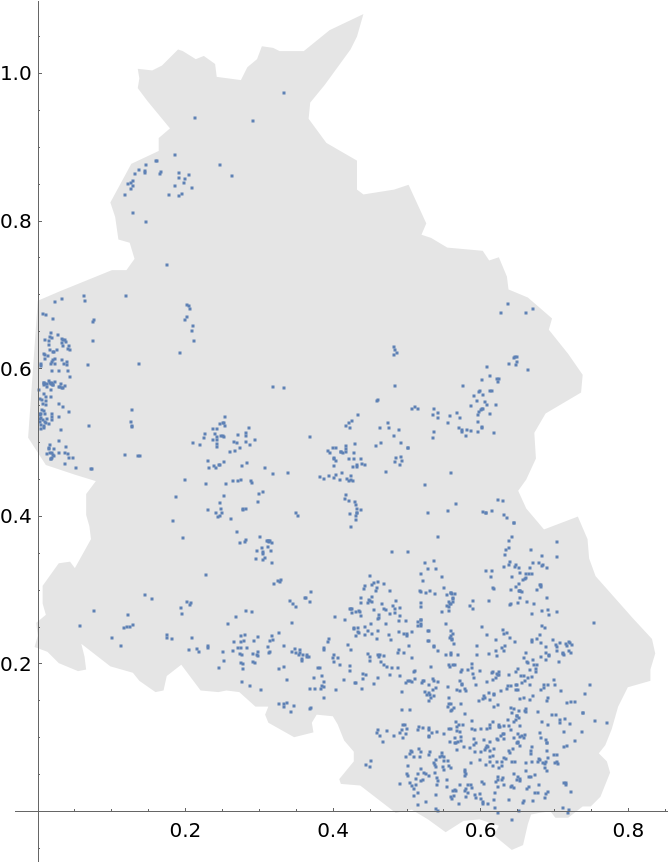Plot the data annotated with gender (3rd annotation) marks:

 In:=Out=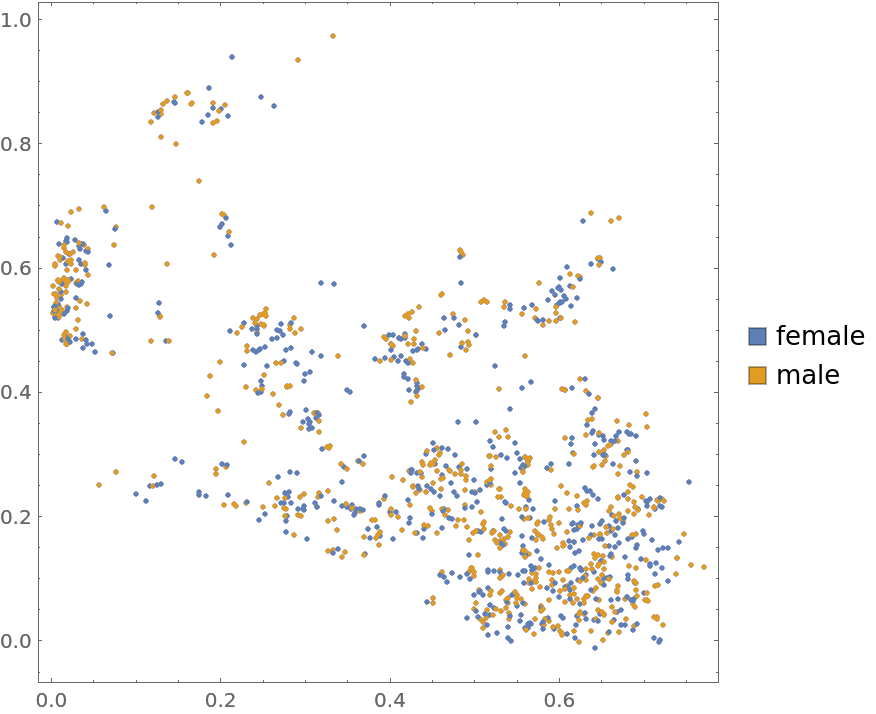Plot the data annotated with type (4th annotation) marks:

 In:=Out=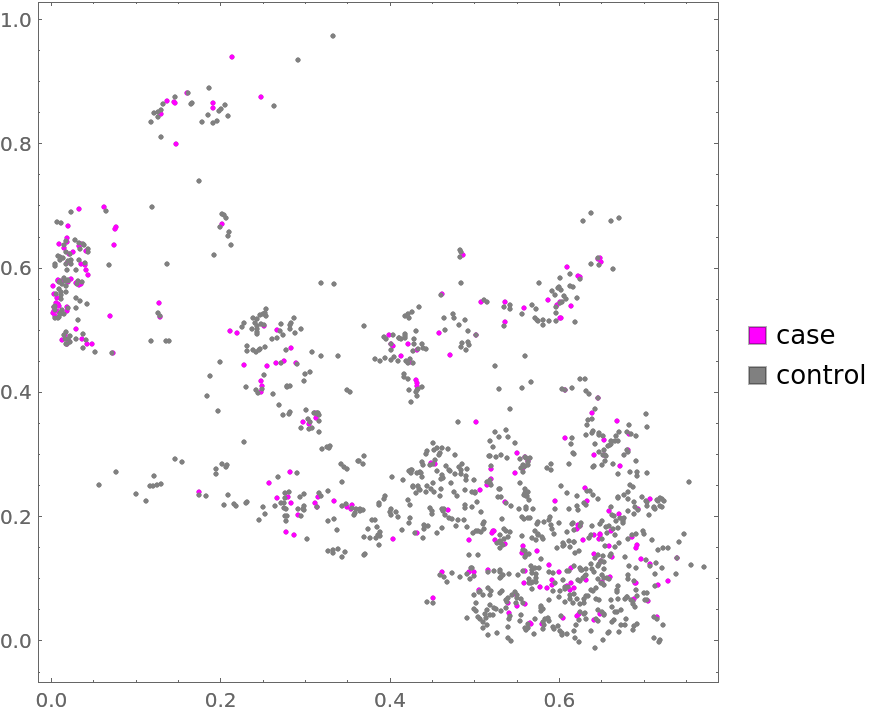### Analysis (3)

Compute probability of finding a point within given radius of an existing point - NearestNeighborG is the CDF of the nearest neighbor distribution:

 In:=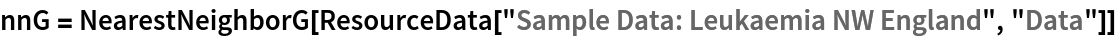Out=In:=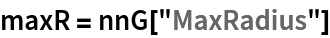Out=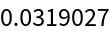In:=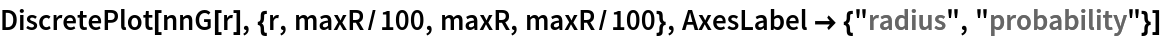Out=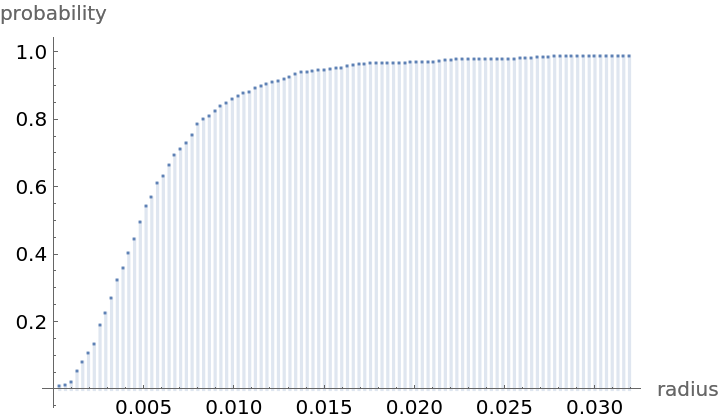Mean distance between a typical point and its nearest neighbor (for positive support distribution can be approximated via a Riemann sum of 1-CDF):

 In:=In:=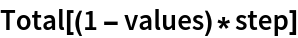Out=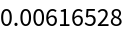Test for complete spacial randomness:

 In:=Out=Gosia Konwerska, "Sample Data: Leukaemia NW England" from the Wolfram Data Repository (2021)